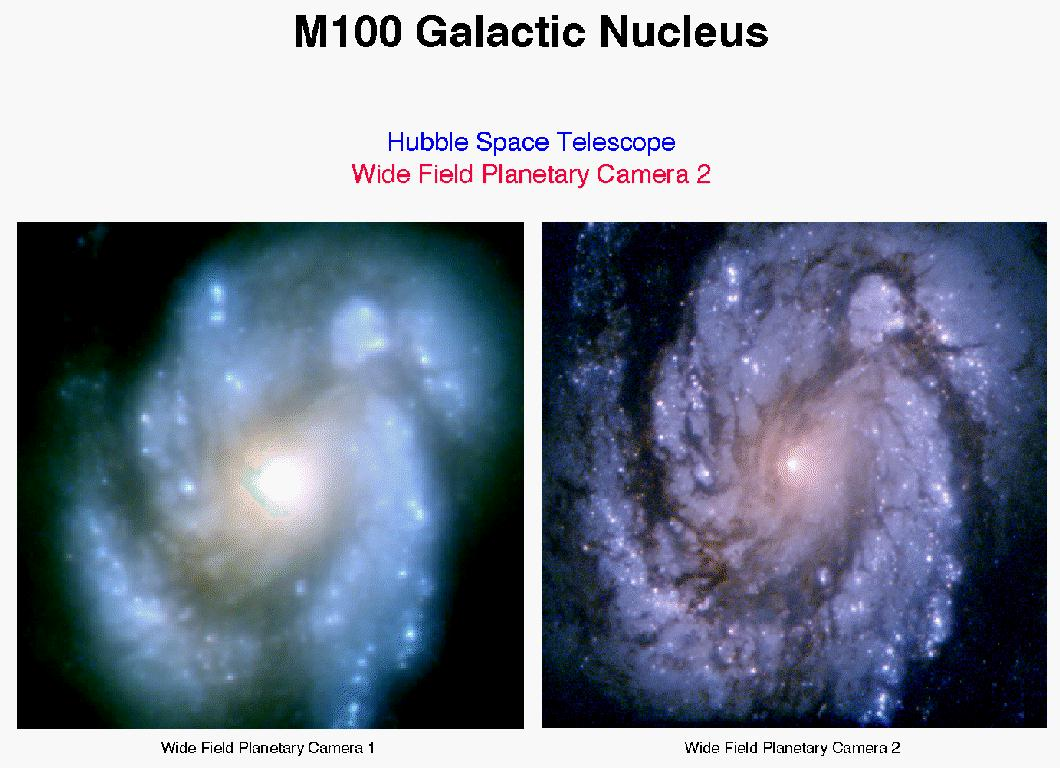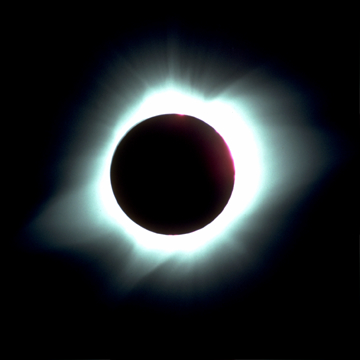## NEXA 380 COSMOLOGIES AND WORLDVIEWS Green and Lea

Physics exercises are not just Math problems.  Every exercise should include a clear, concise discussion of the methods involved and of what you have learned from completing the exercise.

### Assignment #1 Due September 21.

1. In early September we are approaching the autumnal equinox.

a) Observe the sun at sunset on 3 occasions between now and September 21, at the same location. (If sunset is not a convenient time for you, sunrise will do just as well. A mixture of sunrises and sunsets WON'T do!) Stand in exactly the same spot for each observation. Make sketches clearly showing the position of the sun with respect to some foreground features. Be sure the same foreground features appear in each picture.  Note the date and time of your observation on each sketch. (Photographs are also acceptable.)  Discuss how the position of the sun at sunset changes from one observation to the next.

b) Make a sketch showing the position of the Earth in its orbit at this time. Show the position of the Earth, the Sun and the Earth's rotation axis clearly. Also show which way things are moving.

c) Explain your own observations in terms of the model shown in part (b). State clearly how the model is consistent with and explains what you saw.

### Assignment #2 Due October 5.

The Greeks drew heavily on circular motion to construct their cosmologies. Here we use some modern examples to make sure that we understand the concept.

1. Your bicycle wheel has a radius of 1 ft, and you are pedalling so that the wheels turn once per second. How fast is the bicycle moving in feet per second?
Extra credit: how fast is that in miles per hour?

2. The Earth rotates once per day (!) You may assume that the Earth is a sphere with a radius of 6378 km. San Francisco is at Latitude 38°, and so it moves around a circle whose radius is 5026 km.

• a) Everyone: Find how fast we are moving relative to the center of the Earth. (Every speed has to be computed relative to something that is assumed to be at rest.  Here the obvious point is the center of the Earth, which does not participate in the Earth's rotation but rather is on the axis about which the Earth rotates.) Give the answer in km. per hour.
• b) Extra Credit for the scientists: show that the radius of the circle around whose circumference San Francisco moves is 5026 km.
3. Complete the exercise on the handout "Retrograde motion of the planets".

### Assignment #3 Due October 19

Aristotle used lack of parallax to argue against motion of the Earth. Parallax had not been observed by Copernicus' time either, but he was able to show that it should not have been. Only with modern telescopes can we make such accurate measurements. Here are some examples.
1. a) The star Procyon has a parallax of 0.286". What is its distance from the Sun?
b) The star Canopus is 180 light years from the Sun. What is its parallax?

(Reference: Ferris, Chapter 7 and your lecture notes. You'll need the Earth-sun distance from your units handout.)
2. Use the planetary data on your "Ellipses" handout to calculate T2 and a3 and show that all the orbits are consistent with Kepler's 3rd Law. (Note that the numbers given on your sheet are all rounded to two or three significant figures. You can show consistency only to 2 figure accuracy!)
3. Measure the length of the major axis of the ellipse on page 1 of that handout, and the distance between the two foci.  Hence find the eccentricity of the ellipse.  Compare with the values for the planets.  (This should give you an idea of how circular the orbits are, and how careful Kepler had to be to find the differences.)

### Assignment # 4 Due November 9

Newton's discoveries gave us enormously more understanding of the universe. For example:

1. Use Newton's Law of gravitation and your results from problem 2, assignment # 3, to find the mass of the Sun.

The same principles are used in the following exercise:2. The Sun orbits around the center of the galaxy in about 250 million years. The gravitational pull of the material interior to the Sun provides the force to make the Sun move in a circle. Use Newton's Law, as in Problem #1, to find the mass of the galaxy that is interior to the sun. (The distance of the Sun from the center of the galaxy is about 3x1020 m.)

(Material outside the sun has no effect and may be ignored.)

### Assignment #5 Due November 30Almost everyone knows that Einstein discovered the relation E=mc2. But what does that mean in a practical case? For example, how long can the Sun shine?

1. The Sun has a luminosity of 4x1033 erg/s amd a mass m = 2x1033 g.
(The energy unit erg = 1 gram times (1 cm/s)2 and the speed of light is c = 3x1010 cm/s. See Ferris Chapter 10 and class handout.)
(a) By how many grams does its mass decrease per second? Is that a large or a small amount? For example, how many cars is that?
(b) By what percentage does its mass decrease per year? Is that a large or a small number?  If 10% of the Sun's mass is available as fuel, how long can the sun shine before it runs out of fuel?

Einstein's discoveries also changed the way that we view the universe; in particular, he changed our understanding of time.

2.(a) Using graph paper, construct a space-time diagram to illustrate the following events. Use light-days as your unit of length, and days as your unit of time. Be sure your diagram is LARGE enough for you to make measurements easily and accurately.  (Use a full 8x11 page.)

A rocket leaves Earth moving at 4/5 the speed of light. After 2 days Earth-time a radio signal is sent from earth to the rocket. Immediately after the signal is received at the rocket, a reply is sent to Earth.

(b) Use your picture to determine when the rocket receives the signal according to earth-bound clocks, and when the reply is received on Earth.

(c) Use the formula for time dilation to determine how long after departure in rocket time the signal is received at the rocket. Be careful here. For which observers, those on Earth or those on the rocket, do both events occur at the same place?  Use your answer to decide which time interval is the smaller, the one in rocket time or the one in Earth time.

(d) Make another diagram showing events as seen by the rocket crew. (In this diagram the earth moves away at speed (4/5)c.)  Comment!  What have you learned?